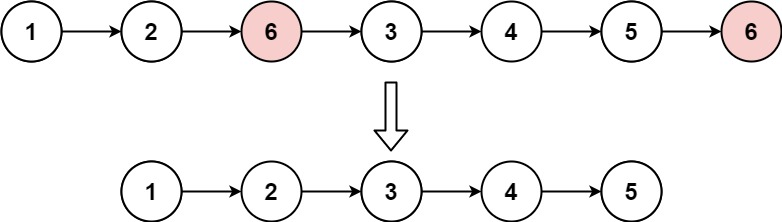## Statement

• Difficulty: Easy
• Tag: `递归` `链表```````输入：head = [1,2,6,3,4,5,6], val = 6

``````

``````输入：head = [], val = 1

``````

``````输入：head = [7,7,7,7], val = 7

``````

• 列表中的节点数目在范围 `[0, 104]`
• `1 <= Node.val <= 50`
• `0 <= val <= 50`

Given the `head` of a linked list and an integer `val`, remove all the nodes of the linked list that has `Node.val == val`, and return the new head.

Example 1:``````Input: head = [1,2,6,3,4,5,6], val = 6
Output: [1,2,3,4,5]
``````

Example 2:

``````Input: head = [], val = 1
Output: []
``````

Example 3:

``````Input: head = [7,7,7,7], val = 7
Output: []
``````

Constraints:

• The number of nodes in the list is in the range `[0, 104]`.
• `1 <= Node.val <= 50`
• `0 <= val <= 50`

## Solution

``````# Definition for singly-linked list.
# class ListNode:
#     def __init__(self, val=0, next=None):
#         self.val = val
#         self.next = next
class Solution:
def removeElements(self, head: ListNode, val: int) -> ListNode:
rt = ListNode()
res = rt